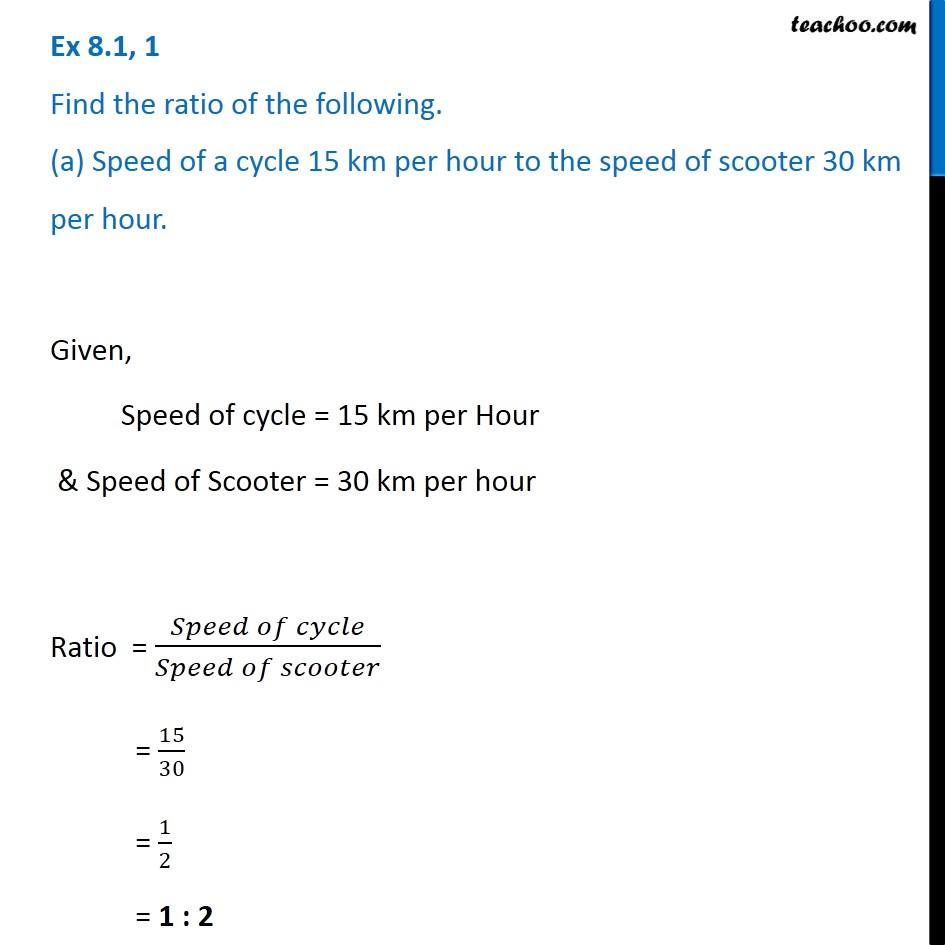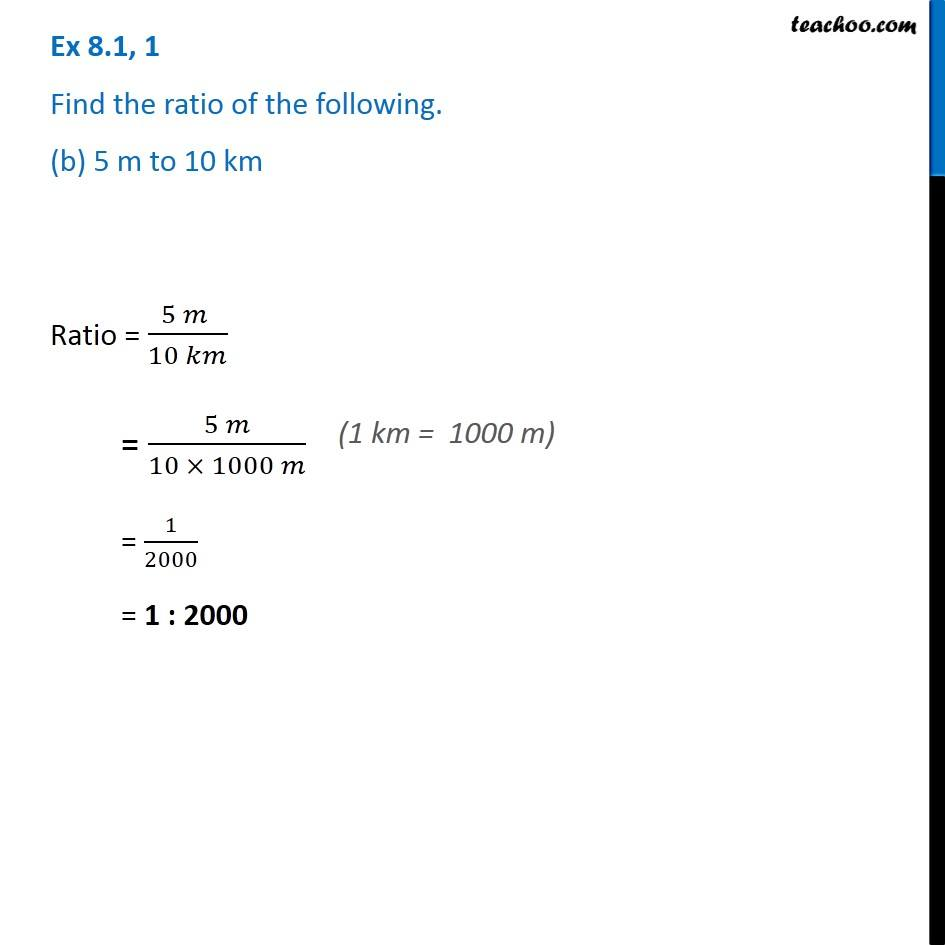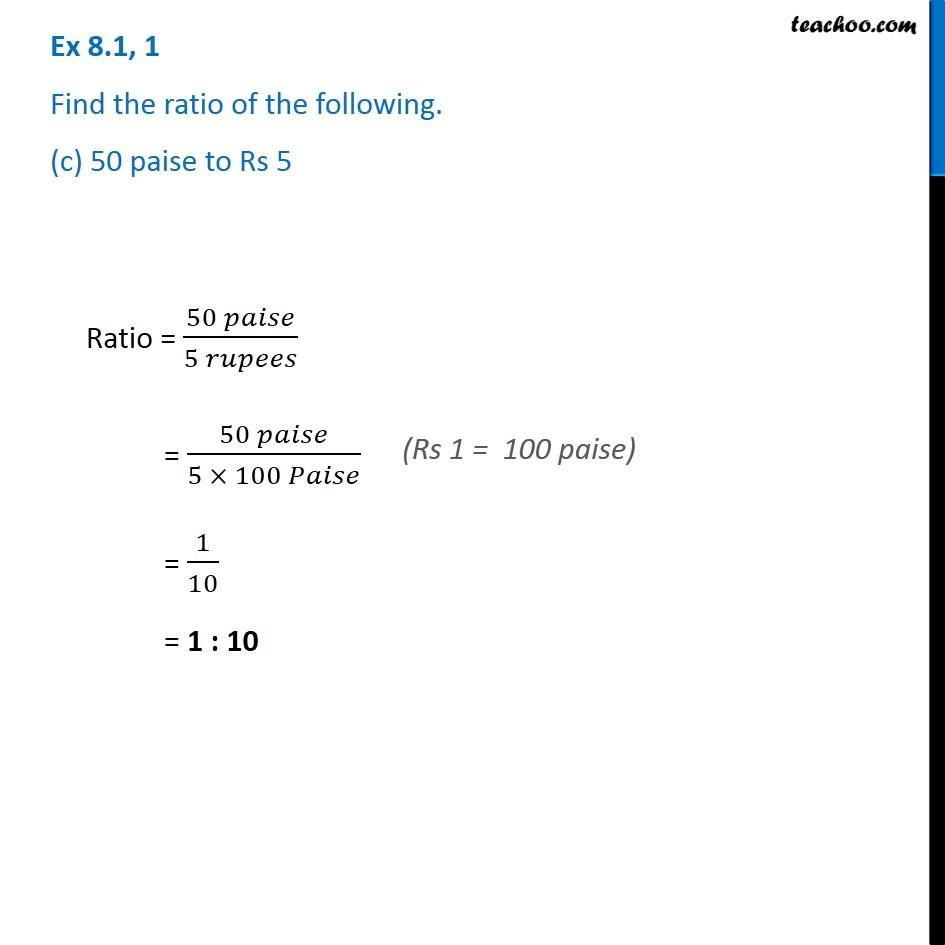Subscribe to our Youtube Channel - https://you.tube/teachoo

1. Chapter 8 Class 8 Comparing Quantities
2. Serial order wise
3. Ex 8.1

Transcript

Ex 8.1, 1 Find the ratio of the following. (a) Speed of a cycle 15 km per hour to the speed of scooter 30 km per hour.Given, Speed of cycle = 15 km per Hour & Speed of Scooter = 30 km per hour Ratio = (𝑆𝑝𝑒𝑒𝑑 𝑜𝑓 𝑐𝑦𝑐𝑙𝑒)/(𝑆𝑝𝑒𝑒𝑑 𝑜𝑓 𝑠𝑐𝑜𝑜𝑡𝑒𝑟) = 15/30 = 1/2 = 1 : 2 Ex 8.1, 1 Find the ratio of the following. (b) 5 m to 10 kmRatio = (5 𝑚 )/(10 𝑘𝑚) = (5 𝑚)/(10 × 1000 𝑚) = 1/2000 = 1 : 2000 (1 km = 1000 m) Ex 8.1, 1 Find the ratio of the following. (c) 50 paise to Rs 5 Ratio = (50 𝑝𝑎𝑖𝑠𝑒)/(5 𝑟𝑢𝑝𝑒𝑒𝑠) = (50 𝑝𝑎𝑖𝑠𝑒)/(5 × 100 𝑃𝑎𝑖𝑠𝑒) = 1/10 = 1 : 10 (Rs 1 = 100 paise)

Ex 8.1